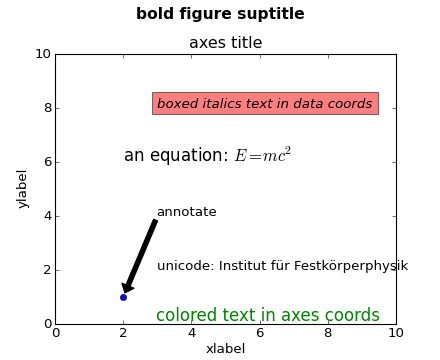#### Previous topic

Working with text

#### Next topic

Text properties and layout

# Text introduction¶

matplotlib has excellent text support, including mathematical expressions, truetype support for raster and vector outputs, newline separated text with arbitrary rotations, and unicode support. Because we embed the fonts directly in the output documents, eg for postscript or PDF, what you see on the screen is what you get in the hardcopy. freetype2 support produces very nice, antialiased fonts, that look good even at small raster sizes. matplotlib includes its own matplotlib.font_manager, thanks to Paul Barrett, which implements a cross platform, W3C compliant font finding algorithm.

You have total control over every text property (font size, font weight, text location and color, etc) with sensible defaults set in the rc file. And significantly for those interested in mathematical or scientific figures, matplotlib implements a large number of TeX math symbols and commands, to support mathematical expressions anywhere in your figure.

# Basic text commands¶

The following commands are used to create text in the pyplot interface

All of these functions create and return a matplotlib.text.Text() instance, which can bew configured with a variety of font and other properties. The example below shows all of these commands in action.

```# -*- coding: utf-8 -*-
import matplotlib.pyplot as plt

fig = plt.figure()
fig.suptitle('bold figure suptitle', fontsize=14, fontweight='bold')

ax.set_title('axes title')

ax.set_xlabel('xlabel')
ax.set_ylabel('ylabel')

ax.text(3, 8, 'boxed italics text in data coords', style='italic',

ax.text(2, 6, r'an equation: \$E=mc^2\$', fontsize=15)

ax.text(3, 2, unicode('unicode: Institut f\374r Festk\366rperphysik', 'latin-1'))

ax.text(0.95, 0.01, 'colored text in axes coords',
verticalalignment='bottom', horizontalalignment='right',
transform=ax.transAxes,
color='green', fontsize=15)

ax.plot(, , 'o')
ax.annotate('annotate', xy=(2, 1), xytext=(3, 4),
arrowprops=dict(facecolor='black', shrink=0.05))

ax.axis([0, 10, 0, 10])

plt.show()
```